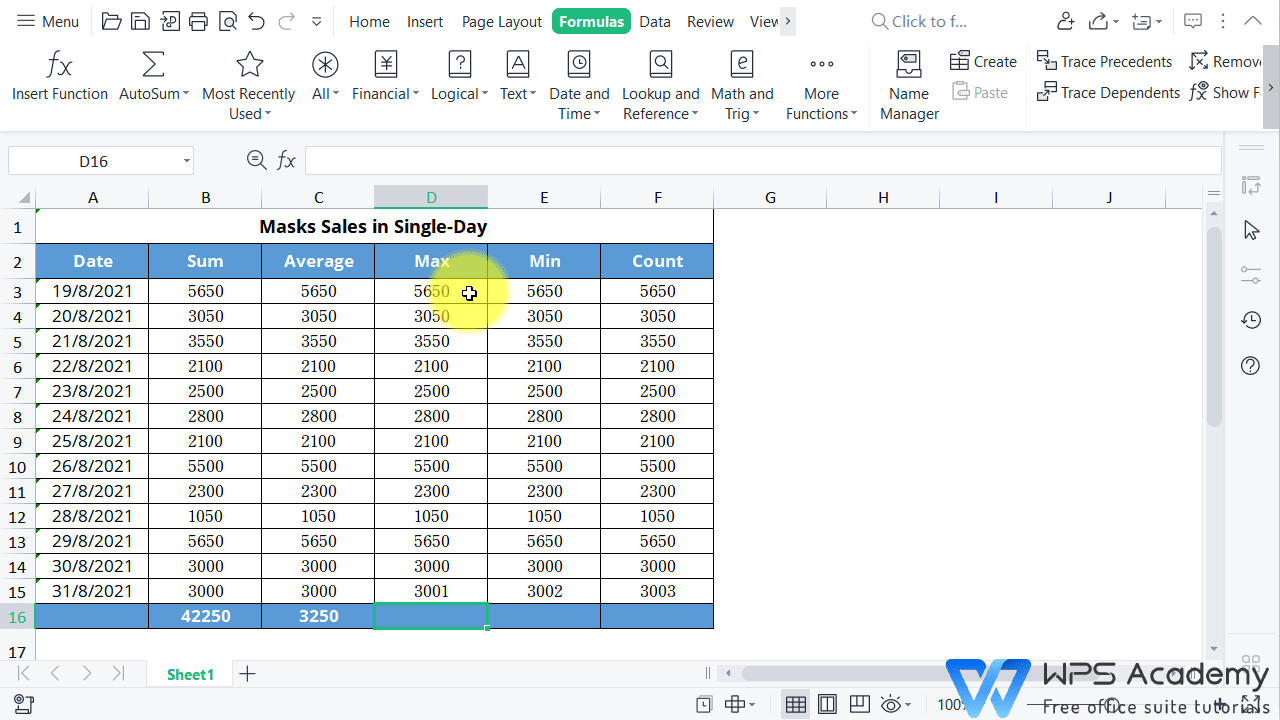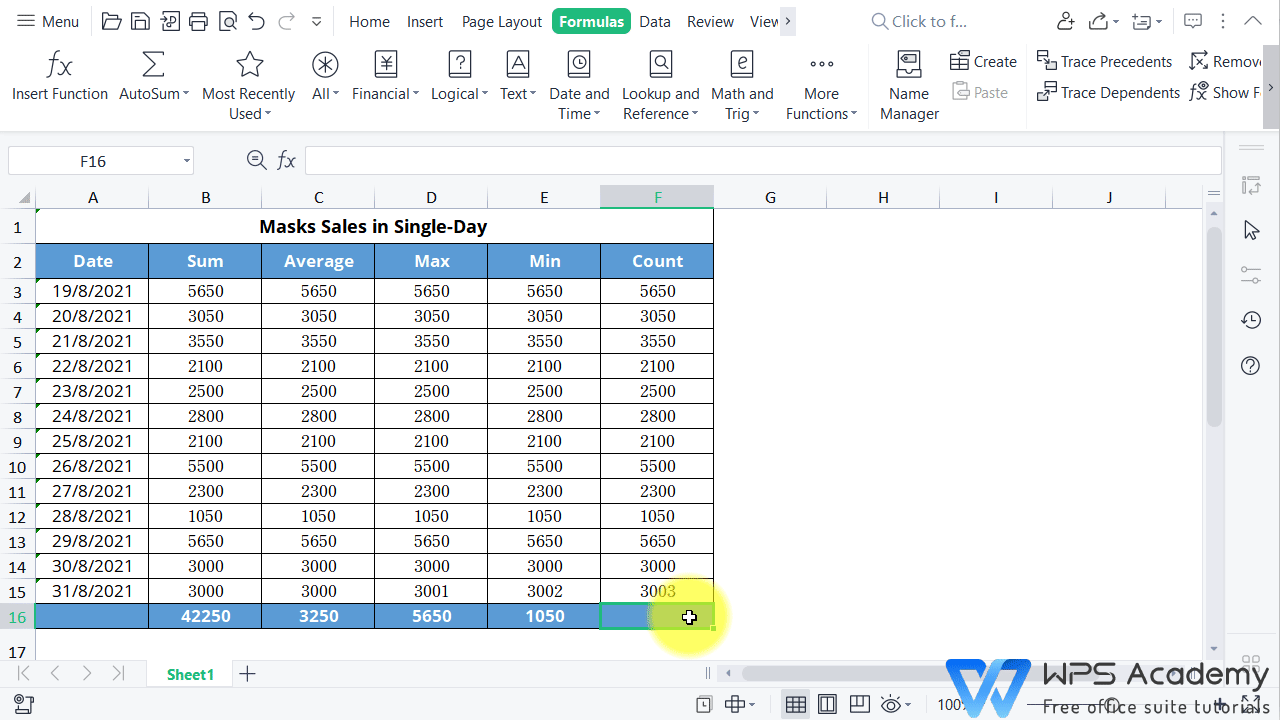# A quick way to calculate sum, average, count, max, and min

Uploaded time: August 26, 2021 Difficulty Beginner

A free Office suite fully compatible with Microsoft OfficeA quick way to calculate sum, average, count, max, and min

# A quick way to calculate sum, average, count, max, and min

Here we have received a sheet on the Masks Sales in Single-Day Sheet from a supermarket. How can we quickly calculate the sum, average, maximum, and daily sales volume during the period from August 19th to 31st?

We can use the AutoSum feature of the WPS Spreadsheet, rather than calculating all data one by one with a calculator, which would be a waste of time. Follow these step.

1. Select the data area, click the AutoSum drop-down button in the Formulas tab.

2. Find the Sum button and click to quickly get the sum value result.

The method is the similiar to findaverages.

1.Select the data area of the table that needs to be averaged.

2. Click the AutoSum drop-down buttonin theFormulas tab.

3. Click the Average button, then you can calculate the average daily sales volume of masks in August.

We can also quickly calculate the maximum sales volume and minimum sales volume of Wal-Mart during this time period.In addition, we can quickly count the number of days that masks have been selling in the supermarket during this time period.

Click the AutoSum drop-down buttonin the Formulas tab, and then the Count button.That's howAutoSum function in WPS Spreadsheet operates. For all, easy our work with WPS Spreadsheet like Microsoft Excel. Did you get it?